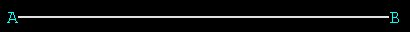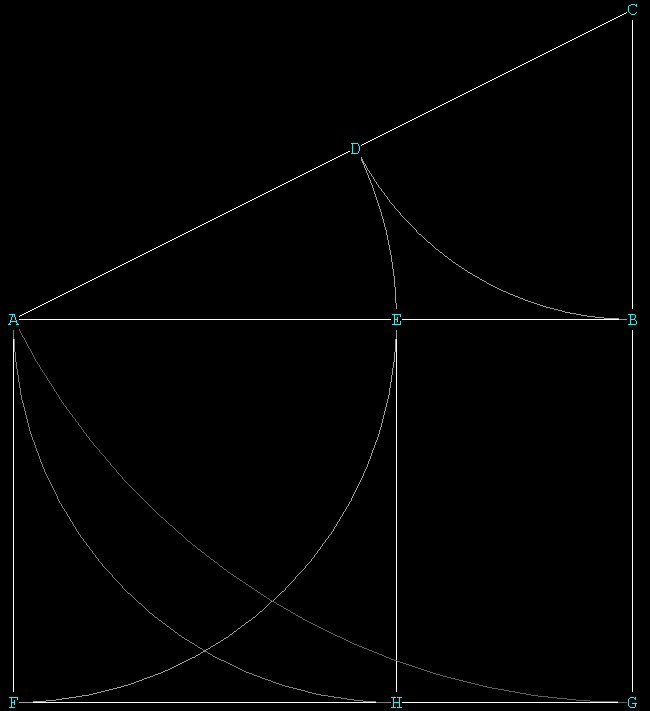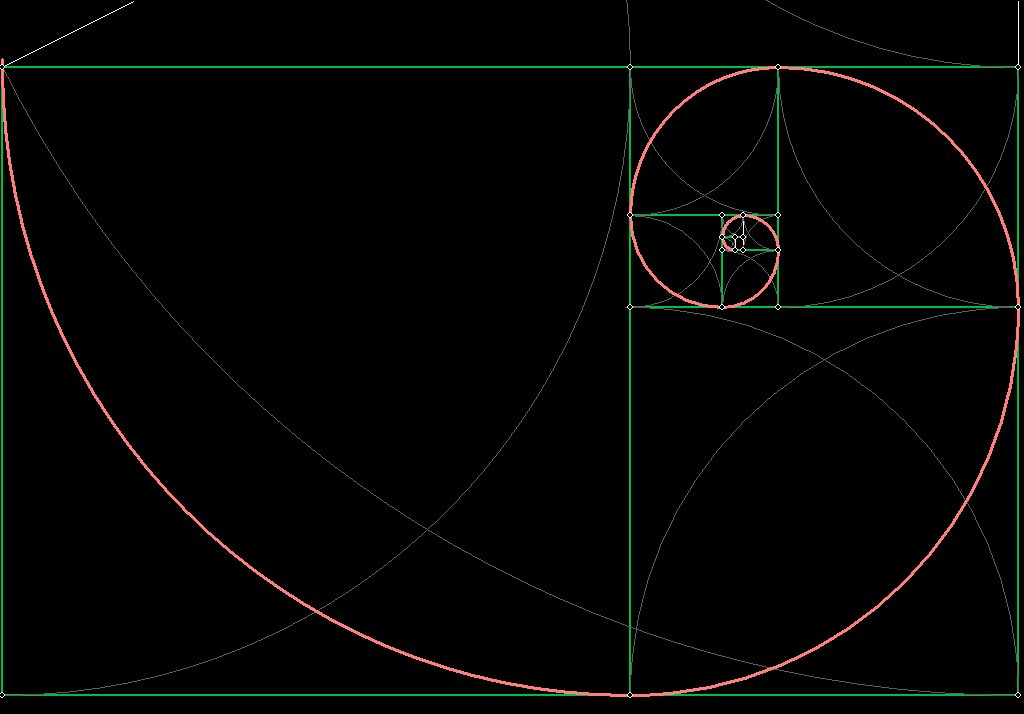A Geometric  Construction of  the Golden Mean Spiral

First we will show how to generate a Golden Mean Spiral geometrically. This is not the only way it can be done, but it is an understandable way. It is also elegant, as the whole thing begins with a line AB of any length:Let's assume that the length of line AB is Unity, or 1.

Now, bisect the line AB and transfer that distance to BC:BC is one-half AB, and is perpendicular to AB.

Connect A to C with the straight-edge. Pin the compass at C and place the marking leg at B. Draw an arc to intersect AC at D.  Now CD = CB.
Now pin the compass at A and place the marking leg at D. Draw an arc to intersect the line AB at E.  Now AE = AD.
The line AB has now been divided in Mean and Extreme Ratio at E:Triangle ABC is a 1, 1/2, \/¯5 / 2 triangle.
AB = 1, BC = 1/2, AC = \/¯5 / 2.
AD = \/¯5 / 2  - 1/2  = 1 / Ø.
Since AD = AE, AE also = 1 / Ø.
EB = 1 / Ø².
AB = (1 / Ø²) + (1 / Ø) = 1.

The point of drawing this triangle is to construct a golden mean rectangle. From the golden mean rectangle we shall build the golden mean spiral. It's pretty cool the way it works out.

Refer to the next drawing below:
With the straight-edge, extend the line CB.
Now, pin the compass at C and place the marking leg at A. Draw an arc to G and extend line CB to intersect the extended line CB, at G.
Since CB = 1/2 and AC = \/¯5 / 2, BG = \/¯5 / 2 - 1/2, or 1 / Ø.
BG therefore = AE.
With the straight-edge, extend the line BA.
Pin the compass at A, and extend the marking leg to E. Draw the circle around A. This circle will intersect the extended line BA, at I.

We are doing this so we can get a perpendicular to the line AB at A, parallel to the line we drew at CG. In geometry you are not allowed to 'eyeball.' You must be precise, so we are making a precise construction.

Now A is precisely in the middle between I and E, because it is the center of the circle with radius AE = AI.

Pin the compass at E, and extend the marking leg to I. Draw an arc.
Pin the compass at I and place the marking leg at E. Draw an arc.
The arc's will intersect at J.
Connect J and A with the straight-edge and extend the line JA so that it intersects the circle. Mark that intersection point F.
This line JA is a geometrically precise perpendicular to the line B, so we know that AF is perpendicular to AB.

Connect F to G to form the line FG. FG is parallel to AB.

Now pin the compass at E with marking leg at A and draw an arc until that arc intersects the line FG at  H.
With the straight-edge, connect E and H.

We have now constructed the Golden Mean Rectangle ABGF.
This is a golden mean rectangle because the 2 long sides, AB and FG, are 1,
and the two short sides AF and BG, are 1 / Ø.In this next drawing I have eliminated all of the unnecessary lines and circles:Not only is ABGF a golden mean rectangle, but AEHF is a square.
I don't want to get too far into the math here, but the cool thing that happens is that EBGH is another golden mean rectangle.
We took the original golden mean rectangle ABGF and made a square within it. What's left over is another golden mean rectangle. That's because each time we make a square from a golden mean rectangle, we are dividing the sides of the golden mean rectangle in Mean and Extreme Ratio.
We can continue this process infinitely by taking squares out of the golden mean rectangles and making more, smaller, golden mean rectangles.
If we use the compass to connect up the diagonals of all of the squares, we can form a golden mean spiral. as shown below:Note that this process can go the other way; instead of creating smaller and smaller rectangles we can make larger and larger ones, so the spiral can grow or contract.